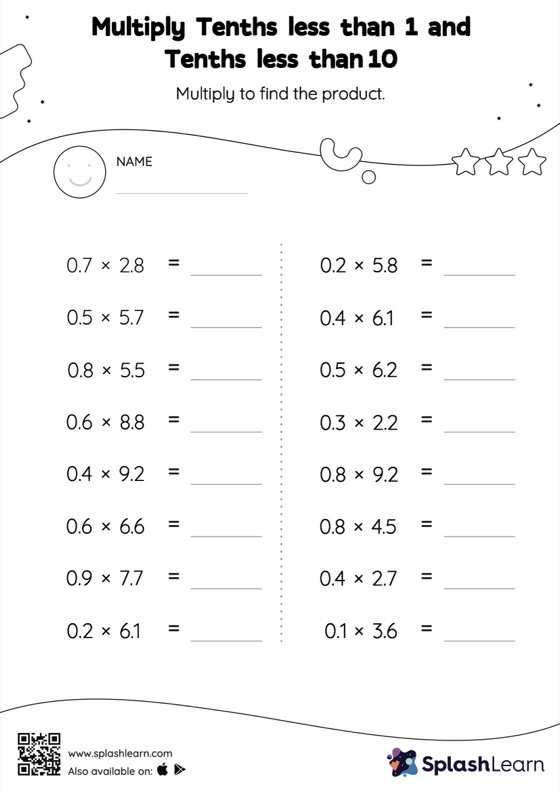# Multiply Tenths less than 1 and Tenths less than 10: Horizontal Multiplication Worksheet

Home > Multiply Tenths less than 1 and Tenths less than 10: Horizontal MultiplicationThis worksheet consists of a set of questions on multiplication where students are required to multiply tenths less than 1 and tenths less than 10. The product of a tenth multiplied by another tenth will have two decimal digits. In the multiply tenths less than 1 and tenths less than 10 worksheet, students practice this idea by multiplying the numbers without considering the decimal points and then adding the decimal point two places from the right in the product. In this worksheet, students practice solving problems written in the horizontal format. How numbers are laid out in a problem affects the method a student employs to solve it. Therefore to develop actual fluency and mastery of multiple strategies, students must practice different formats.